# Carmichael number

A Carmichael number is a composite number named after the mathematician Robert Daniel Carmichael. A Carmichael number$c\$ divides$a^c - a\$ for every integer$a\$. A Carmichael number c also satisfies the congruence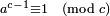$a^{c-1} \equiv 1 \pmod c$, if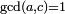$\operatorname{gcd}(a,c) = 1$. The first few Carmichael numbers are 561, 1105, 1729, 2465, 2821, 6601 and 8911. In 1994 Pomerance, Alford and Granville proved that there exist infinitely many Carmichael numbers.

##  Properties

• Every Carmichael number is square-free and has at least three different prime factors
• For every Carmichael number c it holds that c − 1 is divisible by pn − 1 for every one of its prime factors pn.
• Every absolute Euler pseudoprime is a Carmichael number.

##  Chernick's Carmichael numbers

J. Chernick found in 1939 a way to construct Carmichael numbers . If, for a natural number n, the three numbers$6n+1\$,$12n+1\$ and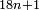$18n+1\$ are prime numbers, the product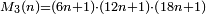$M_3(n) = (6n+1)\cdot (12n+1)\cdot (18n+1)$ is a Carmichael number. This condition can only be satisfied if the number$n\$ ends with 0, 1, 5 or 6. An equivalent formulation of Chernick's construction is that if$m\$,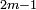$2m-1\$ and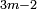$3m-2$ are prime numbers, then the product$m\cdot (2m-1)\cdot (3m-2)$ is a Carmichael number.

This way to construct Carmichael numbers may be extended to$M_k(n)=(6n+1)(12n+1)\prod_{i=1}^{k-2}(9\cdot 2^i n+1) \,$

with the condition that each of the factors is prime and that$n\$ is divisible by 2k − 4.

## Distribution of Carmichael numbers

Let C(X) denote the number of Carmichael numbers less than or equal to X. Then for all sufficiently large X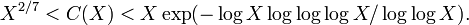$X^{2/7} < C(X) < X \exp(-\log X \log\log\log X / \log\log X) . \,$

The upper bound is due to Erdős(1956) and Pomerance, Selfridge and Wagstaff (1980) and the lower bound is due to Alford, Granville and Pomerance (1994). The asymptotic rate of growth of C(X) is not known.

## References and notes

1. J. Chernick, "On Fermat's simple theorem", Bull. Amer. Math. Soc. 45 (1939) 269-274
2. (2003-11-22) Generic Carmichael Numbers
3. Paulo Ribenboim, The new book of prime number records, Springer-Verlag (1996) ISBN 0-387-94457-5. P.120
4. Paul Erdős, "On pseudoprimes and Carmichael numbers", Publ. Math. Debrecen 4 (1956) 201-206. MR 18 18
5. C. Pomerance, J.L. Selfridge and S.S. Wagstaff jr, "The pseudoprimes to 25.109", Math. Comp. 35 (1980) 1003-1026. MR 82g:10030
6. W. R. Alford, A. Granville, and C. Pomerance. "There are Infinitely Many Carmichael Numbers", Annals of Mathematics 139 (1994) 703-722. MR 95k:11114
7. Richard Guy, "Unsolved problems in Number Theory" (3rd ed), Springer-Verlag (2004) ISBN 0-387-20860-7. Section A13Some content on this page may previously have appeared on Citizendium.